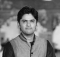## Constructing an array of smaller elements than the corresponding elements based on input array in JavaScriptPublished on 21-Apr-2021 10:24:36
ProblemWe are required to write a JavaScript function that takes in an array of numbers. Our function should construct an output array based on the input array.For each corresponding element our output array should contain the count of number smaller than that number to its right. Finally, we should return ... Read More

## Finding the 1-based index of a character in alphabets using JavaScriptPublished on 21-Apr-2021 10:23:59
ProblemWe are required to write a JavaScript function that takes in a lowercase English alphabet character. Our function should return the character’s 1-based index in the alphabets.ExampleFollowing is the code − Live Democonst char = 'j'; const findCharIndex = (char = '') => {    const legend = ' abcdefghijklmnopqrstuvwxyz';   ... Read More

## Counting number of triangle sides in an array in JavaScriptPublished on 21-Apr-2021 10:23:00
ProblemWe are required to write a JavaScript function that takes in an array of numbers, arr, as the first and the only argument.The task of our function is to count the number of triplets chosen from the array that can make triangles if we take them as side lengths of ... Read More

## Implementing a custom function like Array.prototype.filter() function in JavaScriptPublished on 21-Apr-2021 10:21:13
ProblemWe are required to write a JavaScript function that lives on the prototype Object of the Array class.Our function should take in a callback function as the only argument. This callback function should be called for each element of the array.And that callback function should take in two arguments the ... Read More

## Summing cubes of natural numbers within a range in JavaScriptPublished on 21-Apr-2021 10:20:35
ProblemWe are required to write a JavaScript function that takes in a range array of two numbers. Our function should find the sum of all the cubes of the numbers that falls in the specified range.ExampleFollowing is the code − Live Democonst range = [4, 11]; const sumCubes = ([l, h]) ... Read More

## Calculating and adding the parity bit to a binary using JavaScriptPublished on 21-Apr-2021 10:19:56
Parity BitA parity bit, or check bit, is a bit added to a string of bits to ensure that the total number of 1-bits in the string is even or odd.ProblemWe are required to write a JavaScript function that takes in two parameters, one being the wanted parity (always 'even' ... Read More

## Sorting binary string having an even decimal value using JavaScriptPublished on 21-Apr-2021 10:19:07
ProblemWe are required to write a JavaScript function that takes in a string that contains binary strings of length 3 all separated by spaces.Our function should sort the numbers in ascending order but only order the even numbers and leave all odd numbers in their place.ExampleFollowing is the code − Live ... Read More

## Calculating variance for an array of numbers in JavaScriptPublished on 21-Apr-2021 10:17:36
ProblemWe are required to write a JavaScript function that takes in an array of numbers sorted in increasing order.Our function should calculate the variance for the array of numbers. Variance of a set of numbers is calculated on the basis of their mean.$Mean (M) = ( \sum_{i=0}^{n-1} arr[i])$ / nAnd ... Read More

## Finding the nth power of array element present at nth index using JavaScriptPublished on 21-Apr-2021 10:14:17
ProblemWe are required to write a JavaScript function that takes in an array of numbers. Our function should map the input array to another array in which each element is raised to its 0-based index.And finally, our function should return this new array.ExampleFollowing is the code − Live Democonst arr = ... Read More

## Evaluating a mathematical expression considering Operator Precedence in JavaScriptPublished on 21-Apr-2021 10:13:43
ProblemWe are required to write a JavaScript function that takes in a mathematical expression as a string and return its result as a number.We need to support the following mathematical operators −Division / (as floating-point division)Addition +Subtraction -Multiplication *Operators are always evaluated from left-to-right, and * and / must be ... Read More
Previous 1 ... 3 4 5 6 7 8 9 ... 4825 Next
Advertisements